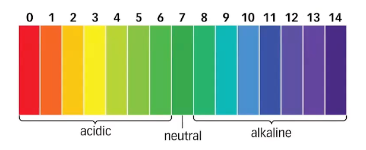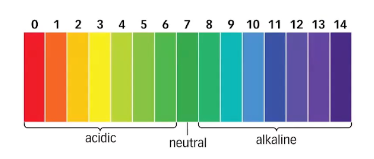Study S2 Science Science- Acids and Alkalis - Geniebook# Acids And Alkalis

In this article, the following are the objectives that will be discussed.

• Define acids.
• Describe the physical properties of acids.
• Define alkalis.
• Describe the physical properties of alkalis.
• Understand the use of the Universal Indicator.
• Describe the chemical properties of acids.

## Acids

Acids are substances which dissociate into H+ ions when dissolved in water.

Here are some examples of acids in our daily life:

• orange juice
• vinegar
• cola drinks

Here are some acids that are commonly found in the laboratory:

• hydrochloric acid
• sulfuric acid
• nitric acid

Here are the physical properties of acids:

• They taste sour due to the presence of proton ions in the solution.
• They have a pH lower than 7.
• They turned blue litmus paper red.
• When acids are dissolved in water, they are able to conduct electricity.

## Alkalis

Alkalis are the substances which dissociate into OH- (hydroxide) ions when dissolved in water.

Here are some examples of alkalis in our daily life:

• toilet cleaner, soap
• baking soda
• ammonia

Here are the physical properties of alkalis:

• They taste bitter and feel soapy.
• They have a pH greater than 7.
• They turned red litmus paper blue.
• They are conductors of electricity.

## pH Level

The pH level indicates how acidic or alkaline a solution is.

• It ranges from 0 to 14, with 0 being the most acidic, 7 being neutral and 14 being the most alkaline.• If the pH level of a substance falls between 4 to 6, it is considered a weak acid.
• If the pH level of a substance falls between 0 to 3, it is considered a strong acid.
• If the pH level of a substance falls between 8 to 10, it is considered a weak alkali.
• If the pH level of a substance falls between 11 to 14, it is considered a strong alkali.

## Universal Indicator

The Universal Indicator helps in the identification of whether a substance is an acidic, neutral or alkaline. Due to the colour changes of the Universal Indicator, it enables one to determine the pH of the substance.• A neutral substance will cause the Universal Indicator to remain green.
• A substance that is weakly acidic will cause the Universal Indicator to change from green to orange/yellow.
• A substance that is strongly acidic will cause the Universal Indicator to change from green to red.
• A substance that is weakly alkaline will cause the Universal Indicator to change from green to blue
• A substance that is strongly alkaline will cause the Universal Indicator to change from green to violet.

## Chemical Properties Of Acids

Acids can undergo three chemical reactions:

1). acid + alkalisalt + water

Example

nitric acid   +   sodium hydroxide   →   sodium nitrate   +   water

hydrochloric acid   +   calcium hydroxide   →   calcium chloride   +   water

sulfuric acid   +   potassium hydroxide   →   potassium sulfate   +   water

2). acid + metalsalt + hydrogen

(Not all metals can react with acids. Unreactive metals, such as copper, silver and gold, do not react with acids.)

Example:

nitric acid   +   aluminium   →   aluminium nitrate   +   hydrogen

hydrochloric acid   +   zinc   →   zinc chloride   +   hydrogen

sulfuric acid   +   magnesium   →   magnesium sulfate   +   hydrogen

• Testing for the presence of hydrogen gas: Place a lighted splint at the mouth of the test tube. If hydrogen gas is present, the lighted splint extinguishes with a ‘pop’ sound.

3). acid   +   carbonate   →   salt   +   water   +   carbon dioxide

Example:

nitric acid   +   calcium carbonate   →   calcium nitrate   +   water   +   carbon dioxide

hydrochloric acid   +   zinc carbonate   →   zinc chloride   +   water   +    carbon dioxide

sulfuric acid   +   copper(II) carbonate   →   copper(II) sulfate   +   water   +   carbon dioxide

• Testing for the presence of carbon dioxide gas: Bubble the gas into limewater. If carbon dioxide gas is present, a white precipitate will be observed.

Revision Questions

Here are some questions for us to look into on the objectives of this article.

Question 1:

Determine whether this statement is true or false.

“Acidic and alkaline solutions taste sour”.

1. true
2. false

Solution:

(B) false

Explanation:

Acidic solutions taste sour while alkaline solutions taste bitter.

Question 2:

Determine whether this statement is true or false.

“Acidic and alkaline solutions are conductors of electricity”.

1. true
2. false

Solution:

(A) true

Explanation:

In acidic and alkaline solutions, there is a presence of free moving ions, which can act as mobile charge carriers, to allow electricity to be conducted.

Question 3:

Which of the following substances is acidic?

1. baking soda
2. vinegar
3. soap
4. toilet cleaner

Solution:

(B) vinegar

Explanation:

Baking soda, soap and toilet cleaner are alkaline substances.

Question 4:

Which of the following substances is not acidic?

1. lime juice
2. cola drink
3. yogurt
4. baking soda

Solution:

(D) baking soda

Explanation:

Lime juice, cola drink and yogurt are acidic substances.

Question 5:

Which of the following is the characteristics of acids?

1. Their pH is lower than 14.
2. They conduct electricity.
3. They cannot react with alkali.
4. They taste bitter.

Solution:

(B) They conduct electricity.

Explanation:

Acids have a pH of less than 7.

Acids react with alkali to form salt and water. This reaction is also known as neutralisation.

Acids taste sour.

Question 6:

Which of the following is the characteristics of alkalis?

1. They cannot conduct electricity.
2. They are tasteless.
3. They turn blue litmus paper red.
4. They can react with acids.

Solution:

(D) They can react with acids.

Explanation:

Alkalis can conduct electricity due to the presence of free moving ions, which can act as mobile charge carriers, to allow electricity to be conducted.

Alkalis have a bitter taste.

Alkalis turn red litmus paper blue.

Question 7:

What is the colour of the Universal Indicator in nitric acid?

1. blue
2. purple
3. red
4. yellow

Solution:

(C) red

Explanation:

Nitric acid is a strong acid. Hence, it turns the Universal Indicator green to red.

Question 8:

Which of the following represents the word equation of acid reacting with alkali?

1. acid + alkali → hydrogen + water
2. acid + alkali → salt + water
3. acid + alkali → salt + water + hydrogen
4. acid + alkali → salt + water + carbon dioxide

Solution:

(B) acid + alkali → salt + water

Explanation:

Acids react with alkalis to form salt and water. This reaction is also known as neutralisation.

Question 9:

Which of the following represents the word equation of acid reacting with carbonate?

1. acid + carbonate → hydrogen + water
2. acid + carbonate → salt + water
3. acid + carbonate → salt + water + hydrogen
4. acid + carbonate → salt + water + carbon dioxide

Solution:

(D) acid + alkali → salt + water + carbon dioxide

Explanation:

Acids react with carbonates to form salt, water and carbon dioxide.

Question 10:

Which of the following represents the word equation of acid reacting with metal?

1. acid + metal → salt + hydrogen
2. acid + metal → salt + water
3. acid + metal → salt + water + hydrogen
4. acid + metal → salt + water + carbon dioxide

Solution:

(A) acid + metal → salt + hydrogen

Explanation:

Acids react metals to form salt and hydrogen gas. However, not all metals react with acids. Unreactive metals, such as copper, silver and gold, do not react with acids.

Question 11:

Which of the following represents the word equation of nitric acid reacting with sodium hydroxide?

1. nitric acid + sodium hydroxide → sodium chloride + water
2. nitric acid + sodium hydroxide → sodium nitrate + water
3. nitric acid + sodium hydroxide → sodium sulfate + water
4. nitric acid + sodium hydroxide → sodium sulfate + hydrogen

Solution:

(B) nitric acid + sodium hydroxide → sodium nitrate + water

Explanation:

Nitric acid is an acid. Sodium hydroxide is an alkali.  Acids react with alkalis to form salt and water.

Question 12:

Which of the following represents the word equation of sulfuric acid reacting with an aluminium carbonate?

1. sulfuric acid + aluminium carbonate → aluminium nitrate + water +    hydrogen
2. sulfuric acid + aluminium carbonate → aluminium sulfate + water + hydrogen
3. sulfuric acid + aluminium carbonate → aluminium nitrate + water + carbon dioxide
4. sulfuric acid + aluminium carbonate → aluminium sulfate + water + carbon dioxide

Solution:

(D) sulfuric acid + aluminium carbonate → aluminium sulfate + water + carbon dioxide

Explanation:

Acids react with carbonates to form salt, water and carbon dioxide.

Continue Learning
Chemical Changes Energy And Work Done
Transfer Of Heat Energy And Its Effects Interactions Within Ecosystems
Electrical Systems Transport System In Living Things
Interactions Through The Application Of Forces Human Sexual Reproductive System
Acids And AlkalisPrimaryPrimary 1Primary 2Primary 3Primary 4Primary 5Primary 6SecondarySecondary 1Secondary 2EnglishMathsScience
Chemical Changes
Energy And Work Done
Transfer Of Heat Energy And Its Effects
Interactions Within Ecosystems
Electrical Systems
Transport System In Living Things
Interactions Through The Application Of Forces
Human Sexual Reproductive System
Acids And AlkalisSecondary 3Secondary 4